Dividing Mixed Numbers - Definition with Examples

The Complete K-5 Math Learning Program Built for Your Child

• 30 Million Kids

Loved by kids and parent worldwide

• 50,000 Schools

Trusted by teachers across schools

• Comprehensive Curriculum

Aligned to Common Core

Dividing Mixed Numbers

Fraction is a part of a whole.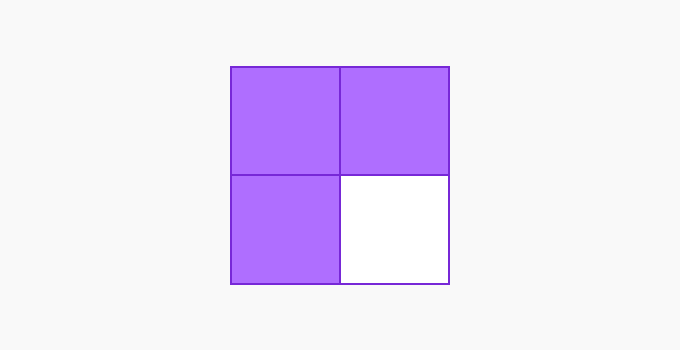The square is divided equally into 4 parts with 3 parts shaded. That is, 3 out of 4 shaded parts are shaded. So, the fraction is  3

The division of 34 by 6 can be shown as: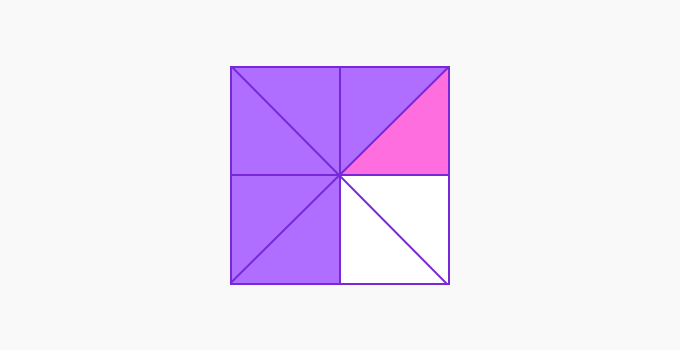The portion shaded in green is one-sixth of the fraction 3.

That is, 34 x 16 = 18 .

Thus, it is one-eighth of the whole square.

When the divisor is a fraction, say  34 ÷ 1, it is clear that there are 3 one-fourths in a three-fourth.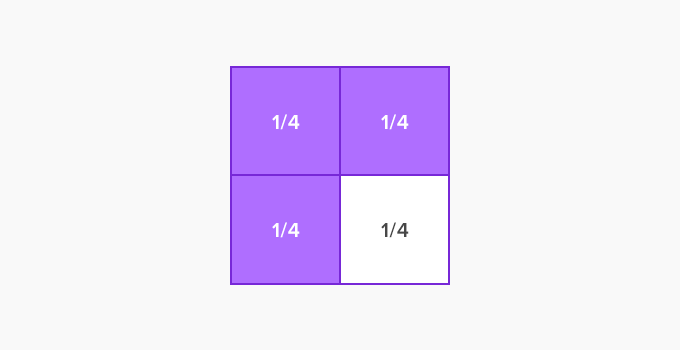So, 34 ÷ 1= 3 .

 To divide a fraction by another, multiply the dividend by the reciprocal of the divisor.

Mixed numbers are combinations of whole numbers and proper fractions.

Examples: 1 12 , 5 34

Note that the unwritten sign between the whole number part and the fractional part is addition, not multiplication!

1 12 glass of milk means one glass and a half glass!

To perform any arithmetic operations on mixed numbers, first, we convert it into an improper fraction- a fraction whose numerator is greater than the denominator.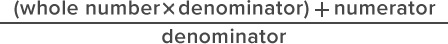To convert an improper fraction to a mixed number, first, divide the numerator by the denominator. The quotient would be the whole number part, and the remainder would be the numerator of the fractional part. The denominator of the mixed number would be the same as that of the improper fraction.

The division rule for fraction remains true for mixed numbers as well.

Example 1: 6 25 ÷ 411

First convert 6 25 into an improper fraction.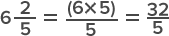25 ÷ 411 325 ÷ 411 =325 x 11885

Now, to convert the improper fraction 885 into a mixed number.

88 ÷ 5=Q17 R3

So, 885 =17 3.

Therefore, 6 25 ÷ 411 = 17 35.

Example 2: Janet has made 12 14 liters of lemonade. She wants to fill them in bottles of capacity 1 34 liters each. How many bottles will she require?

To find the number of bottles required, we need to find the value of 12 14 ÷ 1 3.

First, convert the mixed numbers into improper fractions.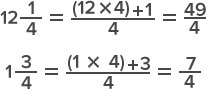The rule for division is to multiply by the reciprocal.

494 ÷ 7= 494 x 7= 7

Therefore, Janet would require 7 bottles to fill the lemonade.

 Fun Facts When you convert an improper fraction to a mixed number and get the remainder zero.  The zero in the numerator makes the fractional part of the mixed number zero!  Thus, the mixed number would be equivalent to the whole number.  48 ÷ 3=Q16 R0 Thus, 48⁄3 = 16.

Won Numerous Awards & Honors